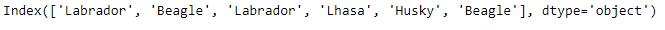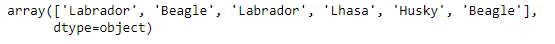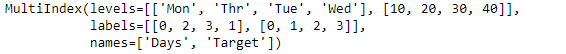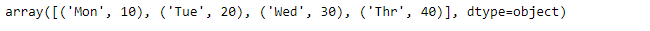# Python | Pandas Index.get_values()

• Last Updated : 17 Dec, 2018

Python is a great language for doing data analysis, primarily because of the fantastic ecosystem of data-centric python packages. Pandas is one of those packages and makes importing and analyzing data much easier.

Pandas` Index.get_values()` function returns the Index data as an numpy.ndarray. It returns one dimensional array for multi-index array.

Syntax: Index.get_values()

Returns : A one-dimensional numpy array of the Index values

Example #1: Use `Index.get_values()` function to return the Index value as a numpy array.

 `# importing pandas as pd``import` `pandas as pd`` ` `# Creating the Index``idx ``=` `pd.Index([``'Labrador'``, ``'Beagle'``, ``'Labrador'``,``                     ``'Lhasa'``, ``'Husky'``, ``'Beagle'``])`` ` `# Print the Index``idx`

Output :let’s use the `Index.get_values()` function to return the Index data as numpy array.

 `# Returns the labels of Index as numpy array``idx.get_values()`

Output :As we can see in the output, the `Index.get_values()` function has returned the index labels as numpy array.

Example #2: Use `Index.get_values()` function on multiindex array.

 `# importing pandas as pd``import` `pandas as pd`` ` `# Creating the MultiIndex object``midx ``=` `pd.MultiIndex.from_arrays([[``'Mon'``, ``'Tue'``, ``'Wed'``, ``'Thr'``],``                   ``[``10``, ``20``, ``30``, ``40``]], names ``=``(``'Days'``, ``'Target'``))`` ` `# Print the MultiIndex object``midx`

Output :Let’s return the Index labels into one dimensional numpy array format.

 `# Convert the multi-index into one``# dimensional numpy array form.``midx.get_values()`

Output :As we can see in the output, even the multi-index has been converted into a one-dimensional array form.

My Personal Notes arrow_drop_up